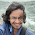### leetcode Question 87: Roman to Interger

Roman to Integer

Given a roman numeral, convert it to an integer.
Input is guaranteed to be within the range from 1 to 3999.

Analysis:
According to the rules from wiki:
• A number written in Arabic numerals can be broken into digits. For example, 1903 is composed of 1 (one thousand), 9 (nine hundreds), 0 (zero tens), and 3 (three units). To write the Roman numeral, each of the non-zero digits should be treated separately. In the above example, 1,000 = M, 900 = CM, and 3 = III. Therefore, 1903 = MCMIII.
• The symbols "I", "X", "C", and "M" can be repeated three times in succession, but no more. (They may appear more than three times if they appear non-sequentially, such as XXXIX.) "D", "L", and "V" can never be repeated.
• "I" can be subtracted from "V" and "X" only. "X" can be subtracted from "L" and "C" only. "C" can be subtracted from "D" and "M" only. "V", "L", and "D" can never be subtracted
• Only one small-value symbol may be subtracted from any large-value symbol.

We can scan the whole string and consider the previous situations then add the relative values to the result.
Details see the code below.

Code:
class Solution {
public:
int romanToInt(string s) {
// Start typing your C/C++ solution below
// DO NOT write int main() function

// 4:IV, 9:IX, 40:XL, 90:XC, 400:CD, 900:CM,
// 1:I, 10:X, 100:C, 1000:M
int res=0;
char pre = ' ';
for(int i=0;i<s.size();i++){
if (s[i]=='M' && pre!='C') {res+=1000;}
if (s[i]=='C' && pre!='X') {res+=100;}
if (s[i]=='X' && pre!='I') {res+=10;}

if (s[i]=='M' && pre=='C') {res+=800;}
if (s[i]=='C' && pre=='X') {res+=80;}
if (s[i]=='X' && pre=='I') {res+=8;}

if (s[i]=='I' ) {res+=1;}

if (s[i]=='V' && pre!='I'){res+=5;}
if (s[i]=='L' && pre!='X'){res+=50;}
if (s[i]=='D' && pre!='C'){res+=500;}

if (s[i]=='V' && pre=='I'){res+=3;}
if (s[i]=='L' && pre=='X'){res+=30;}
if (s[i]=='D' && pre=='C'){res+=300;}

pre = s[i];

}

return res;
}
};


#### 1 comment:

1.This comment has been removed by the author.